# Coulomb's Law

Charles-Augustin de Coulomb was the first to study electrostatic force in the late 1700s. He was trying to understand or determine the strength or size of the force between two stationary charges. He determined, through experimentation, that the electrostatic force increases as the size of the charge increases or as the distance between the charges decreases.

His studies led to Coulomb's Law, which is still used today to determine the size of the electrostatic force between charges. The law states that the magnitude or size of the electrostatic force between 2 point charges is directly proportional to the product of the sizes of the charges and inversely proportional to the square of the distance between them.

In other words, the size of the electrostatic force is affected by two factors - the size of the charge and the distance between the charges. The force will be greater when the charges are larger and the distance between them is less.

Coulomb's Law is represented by the equation:

F=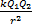,

Where Q1 and Q2, are the sizes of the 2 charges and r is the distance between them. A constant, k, is included and is called the electrostatic constant. k = 9,0 x 109 N.m2.C-2.

The point charges exert a force on one another by means of an electric field.

Examples.

1. Two point-like charges with charges of +3 x 10-9 C and -5 x 10-9 C are 2 m apart. Determine the magnitude of the electrostatic force between them and indicate whether it is an attractive or repulsive force.

F =F =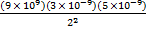F = 3.37 X 10-8 N

The force is attractive, because the charges are opposite, and has a magnitude of 3.37 X 10-8 N.

2. Three point charges exist in a straight line. Details of these charges are shown in the diagram below.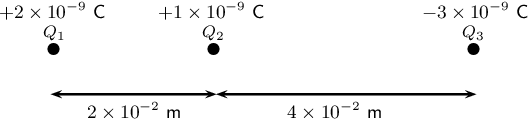Determine the net electrostatic force on Q2 due to the other two charges.

[Tip: Calculate the force on each charge (Q1 and Q3) on Q2 and then add them together, taking into account the direction of the forces - attractive or repulsive.]

F1 =F1 =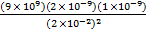F1 = 4.5 X 10-5 N

F1 =F1 =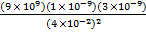F1 = 1.69 X 10-5 N

The force between Q1 and Q2 is repulsive, because they are like charges, and the force between Q2 and Q3 is attractive because they are opposite charges. Therefore, Q2 will move towards Q3.

FR = 4.5 X 10-5 + 1.69 X 10-5 = 6.19 X 10-5 N

The resulting force is 6.19 X 10-5 N to the right.